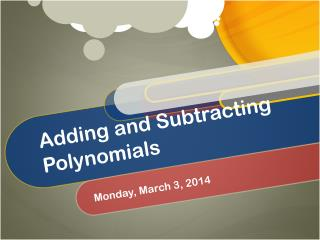DownloadDownload PresentationTélécharger la présentation- - - - - - - - - - - - - - - - - - - - - - - - - - - E N D - - - - - - - - - - - - - - - - - - - - - - - - - - -
Presentation Transcript

1. Adding and Subtracting Polynomials Monday, March 3, 2014

2. Mono- is a prefix meaning “one” • Bi- is a prefix meaning “two” • Tri- is a prefix meaning “three” • Poly- is a prefix meaning “many” • -nomial is a suffix meaning “terms” • Polynomial = Many Terms What are Polynomials?

3. We can perform operations with polynomials (addition, subtraction, multiplication, and division). • When we write polynomials, the standard is to write in descending order of the exponents. • The degree of a polynomial is the highest exponent or sum of the exponents if there are multiple variables. How do we work with Polynomials?

4. Match the polynomial and its degree… • 1. 5p2 – 3 • 2. a3 – 2a2 • 3. 3 – 6n5 – 8n4 • 4. -10x4y3 + 6y3 + 4x4y4 • A. trinomial degree 8 • B. binomial degree 2 • C. trinomial degree 5 • D. binomial degree 3

5. Adding Polynomials is really… Combining Like Terms

6. Subtracting Polynomials is really… Adding the Opposite.

7. Examples: Adding Polynomials (#1) Subtracting Polynomials #(2) (a3 – 2a2) – (3a2 – 4a3) Add the opposite, so… (a3 – 2a2) + (-3a2 + 4a3) Combine like terms… a3 + 4a3 = 5a3 -2a2 + -3a2 = -5a2 5a3 – 5a2 • (5p2 -3) + (2p2 – 3p3) • Identify like terms and combine them. 5p2 + 2p2= 7p2 • Write in descending order of the exponents. • -3p3 + 7p2 -3

8. Note: It can be easier if you put the exponents in order first. (#16) • (-7x5 + 14 – 2x) + (10x4 + 7x + 5x5) • Reorder • -7x5 + 5x5 +10x4 – 2x + 7x + 14 • Combine like terms • -2x5 + 10x4 + 5x + 14

9. Remember, when subtracting, add the opposite, then reorder. (#12) • (8n – 3n4 + 10n2) – (3n2 + 11n4 – 7) • 8n – 3n4 + 10n2 + -3n2 + -11n4 + 7 • -3n4 + -11n4 + 10n2 + -3n2 + 8n + 7 • Combine like terms • -14n4 + 7n2 + 8n + 7

10. Practice makes perfect • Classwork – • Adding and Subtracting Polynomials worksheet (1-30)

11. Practice some more… • Homework – • Adding and Subtracting Polynomials worksheet (1-20) • # 13 and #17-20 are Bonus Problems – Your Exit Ticket is to circle those problems. Please attempt at least one of them.